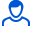Content: teorver_5593.doc (28.00 KB)

Positive responses: 0
Negative responses: 0

Refunds: 05593. x̅=51, n=49, σ=0.5. Based on the sample size n=49, the arithmetic mean x̅в=51 was found. Assuming that X is a normally distributed random variable, find the confidence interval that covers the unknown mathematical expectation a with reliability γ=0.99 if the general standard deviation σ=0.5
Detailed solution. Decorated in Microsoft Word 2003 (Quest decided to use the formula editor)
No feedback yet